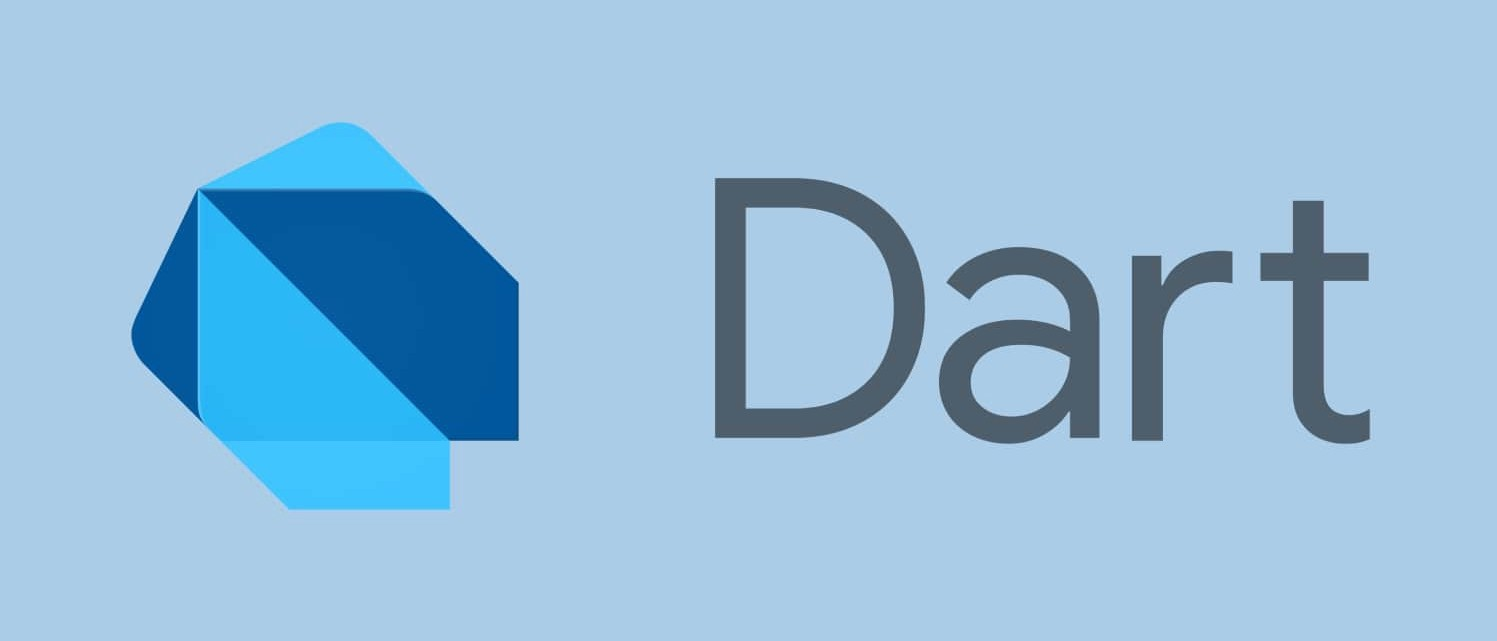### Double in Dart #

In the previous video, We talked about the numbers in Dart. In this video I’ll talk about double in dart.

How to define/declare a double variable ?main.dart
``````double variable_name_2;
``````

How to define/declare a double variable with value ?main.dart
``````double variable_name_2 = 3.5;
``````

Once you declare a variable with it’s type name after that computer will remember that this label is of type integer or double or whatever type you have wrote in the program.

In dart `integer` is refereed as `int`

#### Example 1 #

In this example we will define/declare a variable `x` of double(decimal number) type, after that we will assign some value to `x`.main.dart
``````int main(){
double x;
x = 3.14;
print(x);
}
``````

You can assign some value to the variable at the time of variable declaration also.

#### Example 2 #

Here I defined a variable `x` with the initial value of 3.14.main.dart

``````int main(){
double x = 3.14;
print(x);
}
``````

### Operation on Numeric #

There are various operation which can be performed on Number.

What operation can be performed on Numbers. You can almost perform all the mathematical operation on the number.

## Basic Mathematics Operation #

• Difference (Subtract)
• Product (Multiply)
• Division

#### Sum of Double #main.dart
``````int main(){
double x = 1.20;
double y = 0.30;
double z = x + y;
print(z);   // Result will be 1.50
}
``````

#### Difference of Double #main.dart
``````int main(){
double x = 1.20;
double y = 0.30;
double z = x - y;
print(z);   // Result will be 0.90
}
``````

#### Product of Double #main.dart
``````int main(){
double x = 1.20;
double y = 0.30;
double z = x * y;
print(z);   // Result will be 3.60
}
``````

#### Division of Double #main.dart
``````int main(){
double x = 1.20;
double y = 0.30;
double z = x / y;
print(z);   // Result will be 4.0
}
``````